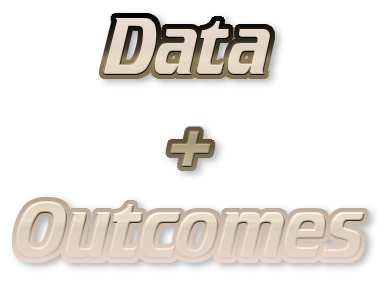# Supervised LearningSupervised learning is concerned with models for predicting the outcome for new data points.

## Models for supervised learning

The following supervised learning models are important:

• Linear models: models that assume the existence of a linear relationship between the independent variables and the outcome.
• Support vector machines: models that deal with non-linear associations by transforming the data to another space via kernel functions.
• Neural networks: models that emulate the interaction of neurons in the nervous system.
• $$k$$-nearest neighbors: a model that classifies a new data point according to its $$k$$ nearest neighbors in the training data.

## Posts on supervised learning

The following posts discuss the use of supervised learning in R.

### An Introduction to Forecasting

0
Forecasting is concerned with making predictions about future observations by relying on past measurements. In this article, I will give an introduction how ARMA, ARIMA (Box-Jenkins), SARIMA, and ARIMAX models can be used for forecasting given time-series data. Preliminaries Before we can talk about models for time-series data, we have to introduce two concepts. The backshift operator Given the time series $$y = \{y_1, y_2, \ldots \}$$, the backshift operator (also called lag operator) is defined as

### Prediction vs Forecasting

Predictions do not always concern the future ...

1
In supervised learning, we are often concerned with prediction. However, there is also the concept of forecasting. Here, I will discuss the differences between the two concepts so that we can answer the question why weather forecasting is not called weather prediction. Predicion and forecasting Prediction is concerned with estimating the outcomes for unseen data. For this purpose, you fit a model to a training data set, which results in an estimator $$\hat{f}(x)$$ that can make predictions for new samples $$x$$.

### Inference vs Prediction

Generative modeling or predictive modeling?

4
The terms inference and prediction both describe tasks where we learn from data in a supervised manner in order to find a model that describes the relationship between the independent variables and the outcome. Inference and prediction, however, diverge when it comes to the use of the resulting model: Inference: Use the model to learn about the data generation process. Prediction: Use the model to predict the outcomes for new data points.

### Linear, Quadratic, and Regularized Discriminant Analysis

3
Discriminant analysis encompasses methods that can be used for both classification and dimensionality reduction. Linear discriminant analysis (LDA) is particularly popular because it is both a classifier and a dimensionality reduction technique. Quadratic discriminant analysis (QDA) is a variant of LDA that allows for non-linear separation of data. Finally, regularized discriminant analysis (RDA) is a compromise between LDA and QDA. This post focuses mostly on LDA and explores its use as a classification and visualization technique, both in theory and in practice.

### Dimensionality Reduction for Visualization and Prediction

0
Dimensionality reduction has two primary use cases: data exploration and machine learning. It is useful for data exploration because dimensionality reduction to few dimensions (e.g. 2 or 3 dimensions) allows for visualizing the samples. Such a visualization can then be used to obtain insights from the data (e.g. detect clusters and identify outliers). For machine learning, dimensionality reduction is useful because oftentimes models generalize better when fewer features are used during the fitting process.

### Interpreting Generalized Linear Models

0
Interpreting generalized linear models (GLM) obtained through glm is similar to interpreting conventional linear models. Here, we will discuss the differences that need to be considered. Basics of GLMs GLMs enable the use of linear models in cases where the response variable has an error distribution that is non-normal. Each distribution is associated with a specific canonical link function. A link function $$g(x)$$ fulfills $$X \beta = g(\mu)$$. For example, for a Poisson distribution, the canonical link function is $$g(\mu) = \text{ln}(\mu)$$.

### Finding a Suitable Linear Model for Ozone Prediction

0
In a previous post, I have introduced the airquality data set in order to demonstrate how linear models are interpreted. In this post, I will start with a basic linear model and, from there, try to find a linear model with a better fit. Data preprocessing Since the airquality data set contains some missing values, we will remove those before we begin to fit models and select 70% of the samples for training and use the remainder for testing:

### Interpreting Linear Prediction Models

0
Although linear models are one of the simplest machine learning techniques, they are still a powerful tool for predictions. This is particularly due to the fact that linear models are especially easy to interpret. Here, I discuss the most important aspects when interpreting linear models by example of ordinary least-squares regression using the airquality data set. The airquality data set The airquality data set contains 154 measurements of the following four air quality metrics as obtained in New York: